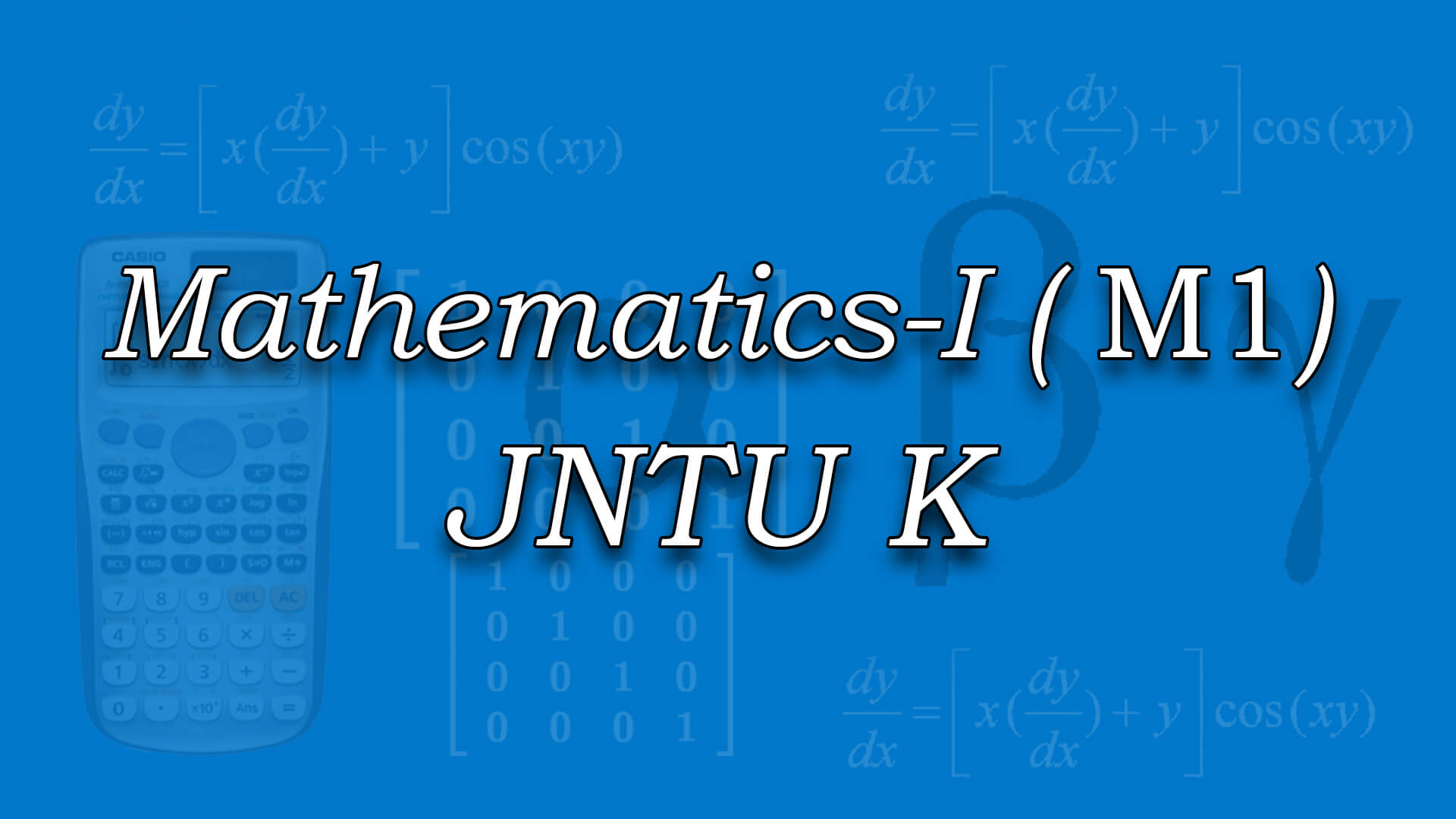## Mathematics-I for JNTUK (R13) by Mr C Srinivas MSc ,B.Ed (Osmania) The course is designed to equip the students with the necessary mathematical skills and techniques that are essential for an engineering course. The skills derived from the course will help the student from a necessary base to develop analytic and design concepts. Code : M1JNTUK Recommended For : B.Tech 1st Year JNTUK

#### After Learning this subject,you should be able to

• Solve linear differential equations of first, second and higher order.
• Determine Laplace transform and inverse Laplace transform of various functions and use Laplace transforms to determine general solution to linear ODE.
• Calculate total derivative, Jacobian and minima of functions of two variables.

## Curriculum 12:00:48   units   |   7

• 1:37:03

UNIT 1
Differential equations of first order and first degree: Exact differential equations, Reducible to exact Part 1, Reducible to exact Part 2, Linear & Bernoulli Equations, Newton?s law of cooling and natural growth and decay, Problems on Newton?s law of cooling, Problems on natural growth and decay, Orthogonal trajectories, Problems on Orthogonal Trajectories Part 1, Problems on Orthogonal Trajectories Part 2, Electrical circuits applications

• 1:45:36

UNIT 2
Linear differential equations of higher order: Linear Differential Equations & Rules for Finding Complimentary Function, Linear Differential Equations & Rules for Finding Complimentary Function 1, Rules for Finding Particular Integral, Rules for Finding Particular Integral 1, Higher order differentional equations Problem 1, Higher order differentional equations Problem 2, Higher order differentional equations Problem 3, Higher order differentional equations Problem 4, Higher order differentional equations Problem 5, Higher order differentional equations Problem 6, Higher order differentional equations Problem 7, Higher order differentional equations Problem 8, Higher order differentional equations Problem 9, Higher order differentional equations Problem 10, Method of variation of parameters

• 2:10:31

UNIT 3
Laplace Transforms: Introduction, transforms of elementary functions, transforms of elementary functions-PART 01, transforms of elementary functions Problems, transforms of elementary functions Problems Part 1, First Shifting theorem, Problems on First Shifting theorem, Properties of laplace transforms, properties of laplace transforms Part 1, Transform of Derivatives & Integrals, Problems transforms of derivatives, transforms of integrals, Multiplication by t power n& Division by t, #NAME?, -division by t .(statements only)

• 1:25:48

UNIT 3.1
Inverse transforms: IT Introduction, Basic Formulas of Inverse Laplace Transform, Problems on Inverse Laplace Transform, Method of Partial fraction Part 1, First Shifting theorem (Table), Problem on First Shifting theorem, Second Shifting Theorem, Inverse Laplace Transform of Derivatives,Integrals, Multiplication & Division by S, IT Convolution theorem, Convolution theorem (without proof), applications to differential equations., applications to differential equations - Part 1

• 2:05:01

UNIT 4
Partial Differentiation: Euler's Theorem, Euler's Theorem Problem 1, Euler's Theorem Problem 2, Total Derivative, Chain Rule of Partial Derivation, Chain Rule of Partial Derivation Problem 1, Chain Rule of Partial Derivation Problem 2, Chain Rule of Partial Derivation Problem 3, Jacobian Determinant, Jacobian Problem 1, Jacobian Problem 2, Functional Dependence and Independence, Maxima and minima of functions of 1 & 2 variables, Lagranges Method, Max and min of functions of 2 & 3 variables using method of Lagrange Multipliers Part 1, Max and min of functions of 2 & 3 variables using method of Lagrange Multipliers Part 2, Max and min of functions of 2 & 3 variables using method of Lagrange Multipliers Part 3, Taylor's Series and Maclaurin's Series, Taylor's Series and Maclaurin's Series Part 1, Taylor's Series and Maclaurin's Series Part 2

• 2:05:26

UNIT 5
First Order Partial Differential Equations: Formation of partial differential equations by elimination of arbitrary constants, Formation of partial differential equations by elimination of arbitrary functions, Formation Of P.D.E By Eliminating arbitrary Constants - Problem 1, Formation Of P.D.E By Eliminating arbitrary Constants - Problem 2, Formation Of P.D.E By Eliminating arbitrary Constants - Problem 3, Formation of PDE By arbitrary functions - Problem 1, Formation of PDE By arbitrary functions - Problem 2, Formation of PDE By arbitrary functions - Problem 3, Formation of PDE By arbitrary functions - Problem 4, Lagranges method to solve the first order linear equations, Lagranges Linear Equation Part 1, Lagranges Linear Equation Part 2, Solutions Of Non Linear Of PDA, The standard type methods to solve the non linear equations Part 1, The standard type methods to solve the non linear equations Part 2, The standard type methods to solve the non linear equations Part 3, Standard Form II Problem, Standard Form II Problem 1, Standard Form II Problem 2, Standard Form III & IV, Standard Form V &VI - Problem 1, Standard Form V &VI - Problem 2

• 0:51:23

UNIT 6
Higher order Partial differential equations: Homogeneous linear partial differential equations with constant coefficients, Homogeneous linear partial differential equations with constant coefficients Part 1, Homogeneous linear partial differential equations with constant coefficients Part 2, Rules for finding complimentary function, rules for finding particular integral